# 数学基础：向量

## 向量的基本运算

### 向量加法：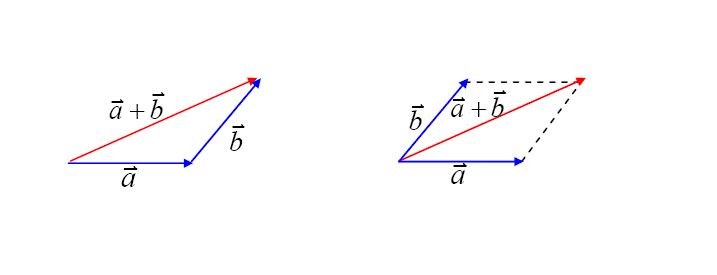### 向量减法：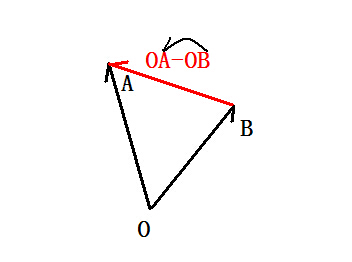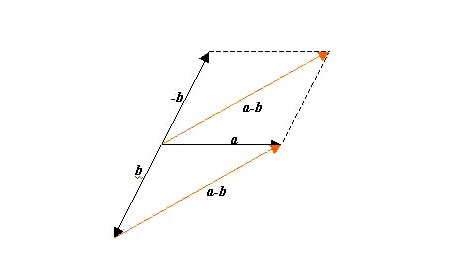### 向量的数乘：

1、当λ>0时：向量λ a⃗  $\vec{a}$的方向与 a⃗  $\vec{a}$的方向相同，其模等于| a⃗  $\vec{a}$|的λ倍，

2、当λ=0时：向量λ a⃗  $\vec{a}$是零向量，即：λ a⃗ 0⃗  $\vec{a}＝\vec{0}$

3、当λ<0时：向量λ a⃗  $\vec{a}$的方向与 a⃗  $\vec{a}$的方向相反，其模等于| a⃗  $\vec{a}$|的|λ|倍，即： |λa⃗ ||λ||a⃗  $|λ\vec{a}|＝|λ||\vec{a}$|；

### 向量的正射影：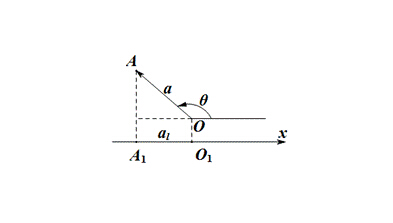OA=a $\vec{OA}=a$在轴l上正射影的坐标记作 al $a_l$，向量a的方向与轴l的正向所成的角为θ，则由三角中余弦定义有：

al=|a|cosθ $a_l=|a|cosθ$

### 向量的内积（数量积、点积）：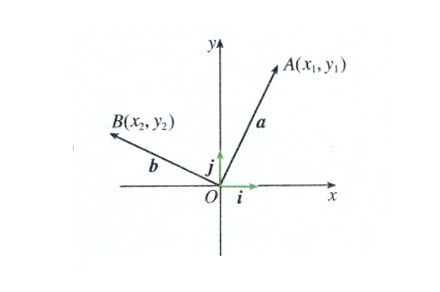ab=|a||b|cos<a,b> $a·b=|a||b|cos$

### 重要性质：

• 如果e是单位向量，则： ae=ea=|a|cos<a,e> $a·e=e·a=|a|cos$
• a⊥b则a·b=0。且a·b=0则a⊥b；
• aa=|a|2 $a·a=|a|^2$ |a|=aa2 $|a|=\sqrt{a·a}$
• cos<a,b> $cos$= ab|a||b| $a·b\over|a||b|$
• |ab||a||b| $|a·b|≤|a||b|$

## 向量与坐标系：

### 数量积的坐标表达式：

ab=a1b1+a2b2 $a·b=a_1b_1+a_2b_2$

a1b1+a2b2=0 $a_1b_1+a_2b_2=0$

### 向量的长度公式：

|a|2=aa=(a1a2)(a1a2)=a21+a22 $|a|^2=a·a=(a_1，a_2 )·(a_1， a_2)=a_1^2+a_2^2$

|a|=a21+a222 $|a|=\sqrt{a_1^2+a_2^2}$

### 向量的距离公式：

AB $\vec{AB}$= (x2x1y2y1) $(x_2-x_1 ，y_2- y_1)$

| AB $\vec{AB}$|= (x2x1)2+(y2y1)22 $\sqrt{(x_2-x_1)^2+(y_2- y_1)^2}$

### 向量的夹角公式：

cos<a,b> $cos$= a1b1+a2b2a21+a222b21+b222 ${a_1b_1+a_2b_2}\over{{\sqrt{a_1^2+a_2^2}·\sqrt{b_1^2+b_2^2}}}$

08-03381
08-0425712-212万+
10-26367
10-08641
07-054170
09-261738
03-083万+
09-20543
09-10124